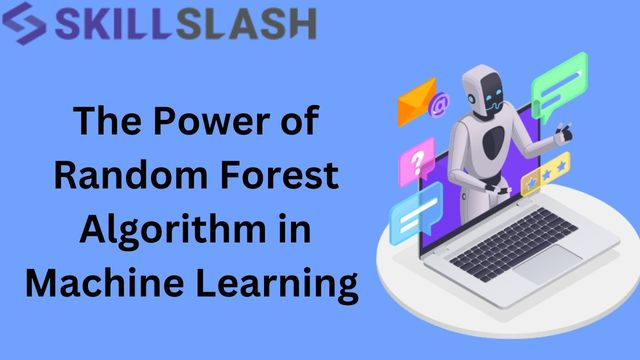News

Oct 26 @ 5:07 amThe Power of Random Forest Algorithm in Machine Le

Introduction

Machine learning is rapidly transforming the way we solve complex problems and make informed data-driven decisions across industries. One of the most widely used machine learning algorithms is the ‘Random Forest Algorithm’. Data scientists and analysts love it because it can handle complex datasets, reduce overfitting, and make accurate predictions.

Here, in this article we dive deep into the Random Forest algorithm, explore real-time applications of the algorithm, and provide  helpful code examples.

Understanding the Random Forest Algorithm

The Random Forest algorithm is a versatile and powerful machine learning technique that has gained immense popularity for its effectiveness in solving both classification and regression problems. In this article, we will explore the key concepts behind the Random Forest algorithm to help you grasp how it works and why it is so widely used.

Ensemble Learning:

Random Forest is a type of ensemble learning algorithm. Ensemble learning is a machine learning approach that combines multiple models to produce a more robust and accurate prediction than individual models. The idea is that by aggregating the predictions of multiple models, any errors or biases in individual models can be mitigated.

Decision Trees:

At the heart of the Random Forest algorithm are decision trees. Decision trees are a type of model that make decisions by splitting data into subsets based on the values of input features. Each split in a decision tree represents a decision or rule, and the tree branches continue to split until a stopping criterion is met, usually involving the purity or impurity of the subsets.

Bootstrapping:

Random Forest employs a technique called bootstrapping. Bootstrapping involves creating multiple random subsets of the dataset by randomly selecting data points with replacements. This results in several training datasets, each slightly different from the original data. Each of these datasets is used to train a different decision tree.

Random Feature Selection:

In addition to bootstrapping, Random Forest also introduces randomness in feature selection. Instead of using all available features when splitting data at each node of a decision tree, it randomly selects a subset of features for each tree. This process reduces the correlation between trees and makes the individual trees more diverse.

Voting Mechanism:

When you want to make a prediction using Random Forest, each decision tree in the forest makes its own prediction. For classification problems, this involves a majority vote, where the class that the majority of decision trees predicts becomes the final prediction. For regression problems, the predictions of individual trees are averaged to make the final prediction.

Real-Time Applications of Random Forest

Random Forest is employed in a variety of fields, including healthcare, finance, image classification, retail and customer segmentation, and environmental science:

-       In healthcare, Random Forest is commonly used to diagnose and prognosis diseases such as diabetes, cancer, and other conditions, taking into account patient data such as age, genetic makeup, clinical history, and more.

-       In finance, Random Forest is employed to detect fraudulent transactions, based on a user’s transaction history and location, as well as other relevant information.

-       In image classification, Random Forest is utilized to identify objects in photographs, recognize handwritten numbers, and even identify faces.

-       In environmental science, Random Forest helps to monitor and predict environmental changes, including deforestation, climate change, and other related issues.

Code Examples

Now, let’s take a look at some Python examples to see how to use Random Forest with the help of Scikit-learn:

Example 1: Classification using Random Forest

 # Import necessary libraries from sklearn.ensemble import RandomForestClassifier from sklearn.datasets import load_iris from sklearn.model_selection import train_test_split from sklearn.metrics import accuracy_score   # Load the Iris dataset data = load_iris() X, y = data.data, data.target   # Split the dataset into training and testing sets X_train, X_test, y_train, y_test = train_test_split(X, y, test_size=0.2, random_state=42)   # Create a Random Forest Classifier clf = RandomForestClassifier(n_estimators=100, random_state=42)   # Train the model clf.fit(X_train, y_train)   # Make predictions y_pred = clf.predict(X_test)   # Calculate accuracy accuracy = accuracy_score(y_test, y_pred) print(f"Accuracy: {accuracy}")

Example 2: Regression using Random Forest

 # Import necessary libraries from sklearn.ensemble import RandomForestRegressor from sklearn.datasets import load_boston from sklearn.model_selection import train_test_split from sklearn.metrics import mean_squared_error   # Load the Boston Housing dataset data = load_boston() X, y = data.data, data.target   # Split the dataset into training and testing sets X_train, X_test, y_train, y_test = train_test_split(X, y, test_size=0.2, random_state=42)   # Create a Random Forest Regressor regressor = RandomForestRegressor(n_estimators=100, random_state=42)   # Train the model regressor.fit(X_train, y_train)   # Make predictions y_pred = regressor.predict(X_test)   # Calculate mean squared error mse = mean_squared_error(y_test, y_pred) print(f"Mean Squared Error: {mse}")

These code examples demonstrate the use of Random Forest for both classification and regression tasks. You can apply similar principles to your specific datasets and problems.

Conclusion

Random Forest is a powerful machine learning algorithm that is renowned for its resilience, adaptability, and capacity to process large datasets. Its applications in various industries, such as healthcare and finance, have demonstrated its value as a predictive modeling tool.

By gaining an understanding of how Random Forest works and using code examples to practice, users can use it to make precise predictions, enhance decision-making capabilities, and address a variety of practical problems. It is an essential tool in the toolbox of any data scientist, providing a reliable and robust way to approach machine learning.

29 Views